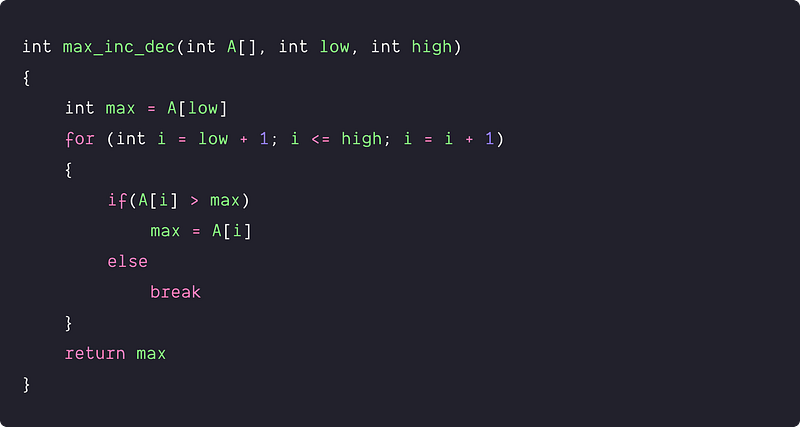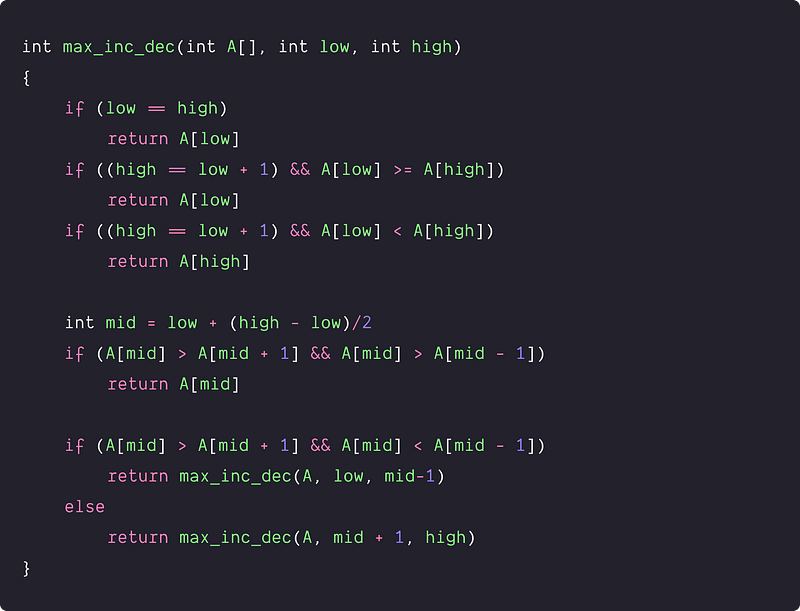# Find the maximum in an array which is first increasing and then decreasing

Key takeaway: This is a good interview problem to learn problem-solving using binary search. The solution idea is intuitive where we modify the binary search algorithm to improve the time complexity.

### Let's understand the problem

You are given an array of integers that is initially increasing and then decreasing, find the maximum value in the array. Hint: keep in mind the corner cases.

Example 1

Input: X[] = [18, 110, 210, 452, 810, 500, 101, 13], Output: 810

Explanation: Array is increasing from start to value 500 and then decreasing. So maximum value in the array = 500

Example 2

Input: X[] = [1, 2, 3, 4, 5], Output: 5

Explanation: Array is sorted in increasing order. So maximum value in the array = 5

### Discussed solution approaches

• A brute force approach using linear search
• An efficient approach  using binary search

### A brute force approach  using linear search

Solution Idea and Steps

A simple approach is to traverse the array linearly and find the maximum.

• We initialize a variable max with the first element to track the maximum element.
• Now we traverse from the second element to the last element using a loop.
• When we find a value A[i] greater than max, we update max with A[i] and continue this process until the values in the array keep increasing. In other words, our max value is at the peak, and we reach thereby updating the variable max with A[i].
• We need to stop at the peak point because, after that, the array values start decreasing. In other words, when we first time find some A[i] < max, we break the loop and return the value stored in the max.

Solution PseudocodeTime and space complexity analysis

The worst-case occurs when the array is sorted in increasing order, and we need to traverse each element till the end. So worst-case time complexity = O(n). What would be the best and average-case time complexity? Think! We are using a constant number of variables, so space complexity = O(1)

But the critical question is: how can we improve the time complexity? How do we use the ‘first increasing and then decreasing’ property given in the input to enhance the solution's efficiency? Think!

### An efficient approach  using binary search

Solution Idea and Steps

In the ‘first increasing and then decreasing’ array, the max value in the array would be the peak point or a point after which the values started decreasing. One intuition of the efficient solution is — can we apply the idea of binary search to find such a specific point because elements in the array are sorted in a particular order? But how do we use the concept of binary search? Think! Here are the observations to modify the standard binary search algorithm:

• If the mid element is greater than both of its adjacent elements, then the value at mid is the maximum. In other words, the array is decreasing after the midpoint i.e. if (A[mid] > A[mid-1] && A[mid] > A[mid+1]), then we return A[mid].
• If the mid element is greater than its next element and smaller than the previous element then maximum lies on the left sub-array i.e. if (A[mid] > A[mid+1] && A[mid] < A[mid-1]) then we search max in the left subarray. In other words, we are on the decreasing side of the array and the peak will be somewhere between low to mid - 1. Think!
• If the mid element is smaller than its next element and greater than the previous element then maximum lies on the right sub-array i.e. if (A[mid] < A[mid+1] && A[mid] > A[mid-1]) then we search max in the right subarray.In other words, we are on the increasing side of the array and the peak will be somewhere between mid + 1 to high. Think!

Solution PseudocodeTime and space complexity analysis

Since we are using recursive binary search, so the time complexity of the above program would be O(log n). The space complexity depends on the size of the call stack which is equal to the height of the recursion tree. So space complexity = O(logn). Think!

### Critical ideas to think!

• How do we implement the efficient approach using the iterative binary search?
• Does the binary search solution is correct If the array contains duplicates? If not then, how do we modify the solution to work for duplicate elements?
• How to modify the above code to cover edge cases when the array is purely increasing or purely decreasing?
• How do we modify the above code if we have to find a minimum value when the array is first decreasing and increasing?

### Comparisons of time and space complexities

• Linear search: Time = O(n), Space = O(1)
• Recursive binary search: Time = O(logn), Space = O(logn)

### Coding problems to practice using binary search

Thanks to Navtosh Kumar for his contribution in creating the first version of the content. Please write comments if you find an error or you want to share more insights about the topic. Enjoy learning, Enjoy coding, Enjoy algorithms!

Get well-designed application and interview centirc content on ds-algorithms, machine learning, system design and oops. Content will be delivered weekly.